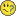# New to QlikView

Discussion board where members can get started with QlikView.

Highlighted
Contributor III

## Lookup equivalent value from expression result

I have a mapping table in my script which equates points to grades. The table is approx. 100 rows, and starts as follows:

45D
46D
47D
48C
49C

In my qvw, most of my expressions are built using the points (obviously, as I need a numerical value for my calculations). However, in some of my tables, I would like to show the grade equivalent.

I have one table that shows the average of all candidates points against a yearly dimension, e.g.:

2012=AVG(Points)
2013=AVG(Points)
2014=AVG(Points)

Obviously the value in the Points column changes according to different selections that are made.

How can I show the equivalent grade too?

Thanks for any suggestions.

Tags (1)
8 RepliesMVP

## Re: Lookup equivalent value from expression result

Something like the attached?

Contributor III

## Re: Lookup equivalent value from expression result

Hi Sunny,

This seems like a great start. But what if I don't want to include the 'Year' dimension in the expression? Ideally I'd like it to be separate from that as I have a LOT of data, and I can't easily create the inline table that you've used in your example.

So to use your example script, my 'Table' has

Year,

Points,

etc

etc

So if I created a straight table, then I would see what the points/grade equivalent is.

It's where I'm using the 'AVG' function that it all goes wrong.

Any ideas?

Honored Contributor

## Re: Lookup equivalent value from expression result

Hi Jessica,

I haven't test this expression so it might not work straight out of the box but what about this expression?

=FirstSortedValue({\$<Points = {"<=\$(=Avg(Points))"} >} Grade, -Points)

Contributor III

## Re: Lookup equivalent value from expression result

Hi Andrew,

It's not working quite as it should - in a couple of instances I get no result at all (I think because the values are averages (i.e. they have decimal numbers), whereas my equivalence table is on whole numbers only).

But on the one instance it does give me a result, it's not what I would expect - e.g. for an average point score of 46, it gives me a result of 'C' (when it should be a 'D').MVP

## Re: Lookup equivalent value from expression result

Can you may be create a sample which shows us what you are looking to do?

Honored Contributor

## Re: Lookup equivalent value from expression result

Hello Jessica,

Something's a bit odd. This formula with the inequality hard written as <=46 returns a 'D'  with the five lines of your mapping table loaded into my qvw.=FirstSortedValue({\$<Points = {"<=46"} >} Grade, -Points)

=================================================

I've just realised something (I can be very slow). My method won't work on your mapping table as that table will not exist in the data model after the reload.

Sorry!Contributor III

## Re: Lookup equivalent value from expression result

Got you - no worries at all. Thanks for the suggestions anyway!

Contributor III

## Re: Lookup equivalent value from expression result

Hi Sunny,

Unfortunately under a bit of time pressure so haven't managed to create a sample file.

As a temporary fix for now (so that I can send a demo to our client later today), I have used a very convoluted 'if' statement, which does work - but probably isn't great for overall efficiency!

IF(Avg(Points)>=89,'E9',IF(Avg(Points)>=88,'E8',IF(Avg(Points)>=87,'E7',IF(Avg(Points)>=86,'E6',IF(Avg(Points)>=85,'E5',IF(Avg(Points)>=84,'E4',IF(Avg(Points)>=83,'E3',IF(Avg(Points)>=82,'E2',IF(Avg(Points)>=81,'E1',IF(Avg(Points)>=80,'D9',IF(Avg(Points)>=79,'D8',IF(Avg(Points)>=78,'D7',IF(Avg(Points)>=77,'D6',IF(Avg(Points)>=76,'D5',IF(Avg(Points)>=75,'D4',IF(Avg(Points)>=74,'D3',IF(Avg(Points)>=73,'D2',IF(Avg(Points)>=72,'D1',IF(Avg(Points)>=71,'C9',IF(Avg(Points)>=70,'C8',IF(Avg(Points)>=69,'C7',IF(Avg(Points)>=68,'C6',IF(Avg(Points)>=67,'C5',IF(Avg(Points)>=66,'C4',IF(Avg(Points)>=65,'C3',IF(Avg(Points)>=64,'C2',IF(Avg(Points)>=63,'C1',IF(Avg(Points)>=62,'B9',IF(Avg(Points)>=61,'B8',IF(Avg(Points)>=60,'B7',IF(Avg(Points)>=59,'B6',IF(Avg(Points)>=58,'B5',IF(Avg(Points)>=57,'B4',IF(Avg(Points)>=56,'B3',IF(Avg(Points)>=55,'B2',IF(Avg(Points)>=54,'B1',IF(Avg(Points)>=53,'A9',IF(Avg(Points)>=52,'A8',IF(Avg(Points)>=51,'A7',IF(Avg(Points)>=50,'A6',IF(Avg(Points)>=49,'A5',IF(Avg(Points)>=48,'A4',IF(Avg(Points)>=47,'A3',IF(Avg(Points)>=46,'A2',IF(Avg(Points)>=45,'A1',IF(Avg(Points)>=44,'P8.9',IF(Avg(Points)>=43,'P8.8',IF(Avg(Points)>=42,'P8.7',IF(Avg(Points)>=41,'P8.6',IF(Avg(Points)>=40,'P8.5',IF(Avg(Points)>=39,'P8.4',IF(Avg(Points)>=38,'P8.3',IF(Avg(Points)>=37,'P8.2',IF(Avg(Points)>=36,'P8.1',IF(Avg(Points)>=35,'P7.9',IF(Avg(Points)>=34,'P7.8',IF(Avg(Points)>=33,'P7.7',IF(Avg(Points)>=32,'P7.6',IF(Avg(Points)>=31,'P7.5',IF(Avg(Points)>=30,'P7.4',IF(Avg(Points)>=29,'P7.3',IF(Avg(Points)>=28,'P7.2',IF(Avg(Points)>=27,'P7.1',IF(Avg(Points)>=26,'P6.9',IF(Avg(Points)>=25,'P6.8',IF(Avg(Points)>=24,'P6.7',IF(Avg(Points)>=23,'P6.6',IF(Avg(Points)>=22,'P6.5',IF(Avg(Points)>=21,'P6.4',IF(Avg(Points)>=20,'P6.3',IF(Avg(Points)>=19,'P6.2',IF(Avg(Points)>=18,'P6.1',IF(Avg(Points)>=17,'P5.9',IF(Avg(Points)>=16,'P5.8',IF(Avg(Points)>=15,'P5.7',IF(Avg(Points)>=14,'P5.6',IF(Avg(Points)>=13,'P5.5',IF(Avg(Points)>=12,'P5.4',IF(Avg(Points)>=11,'P5.3',IF(Avg(Points)>=10,'P5.2',IF(Avg(Points)>=9,'P5.1',IF(Avg(Points)>=8,'P4.9',IF(Avg(Points)>=7,'P4.8',IF(Avg(Points)>=6,'P4.7',IF(Avg(Points)>=5,'P4.6',IF(Avg(Points)>=4,'P4.5',IF(Avg(Points)>=3,'P4.4',IF(Avg(Points)>=2,'P4.3',IF(Avg(Points)>=1,'P4.2',IF(Avg(Points)>=0,'P4.1'

))))))))))))))))))))))))))))))))))))))))))))))))))))))))))))))))))))))))))))))))))))))))))

Probably Qlikview's worst expression ever, but it'll have to do for now...